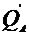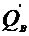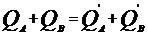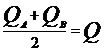Electricity & Megnetism

# The contact electrification

Consider two conducting spheres A and B, one electrified (A) and one neutral (B).

When we place the positively charged sphere A in contact with the sphere B, the former attracts part of the electrons from B. Thus, A remains positively charged, but with a smaller charge, and B, which was neutral, becomes electrified with a positive charge.
This is the simplest way to electrify a body. When two bodies are touching or connected by wires, electrons can pass from one to the other. For this type of electrification to take place, bodies and wires must be conductors, not insulators.

We can then say that if a negatively electrified body (with an excess of electrons) is leaning against another, neutral, part of its electrons will pass to this one, which will also be negatively electrified.

If the first body were positively charged (short of electrons), it would withdraw electrons from the neutral body, so that both would be short of electrons and therefore positively electrified.

According to the principle of conservation of electrical charges, the algebraic sum of negative electrical charges and positive charges, assuming the system is electrically isolated, is constant.
For example:
Suppose that two bodies A and B, isolated from any external influence, initially have charges QA and QB, respectively. If they are brought into contact, there will be an exchange of electrical charges between them, so that after some time the respective charges will beand. According to the principle of conservation of charge, we can write:In contact electrification, the exchange of charges depends on the dimensions of the conductors.
If we consider that the bodies have the same dimensions and the same shape, being, for example, spheres of the same radius, after contact they will present equal charges.
The mathematical equation that represents the balance of charges, in identical conductors, is:Where Q is the electrostatic equilibrium charge, that is, the charge common to the two bodies after contact.